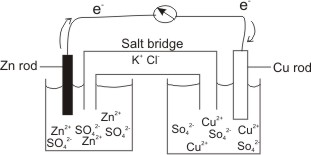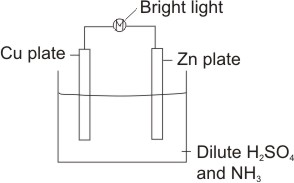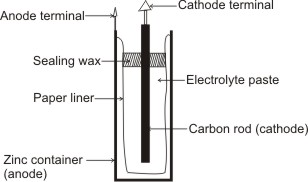## Voltaic or Galvanic Cell

When two electrode potentials are joined together by a wire, an electrochemical, voltaic or galvanic cell is produced.

Example, a Zn metal in a Zinc salt solution joined to a Cu metal in a Cu salt by a conducting wire.From the electrochemical series, it is clear that Cu2+ is more reduced than Zn2+.

This means that Cu2+ will acquire electrons and become reduced more readily than Zn2+. Hence by connecting their electrode potentials, the Zn electrode will lose electrons and become oxidized.

Zn(s) → Zn2+(aq) + 2e-(aq)

These electrons will flow from the Zn rod through the conducting wire into the Cu electrode, where they are picked up by the Cu2+ to become reduced.

Cu2+(aq) + 2e-(aq) → Cu(s)

Thus electricity is generated. The U-tube, which connects the two electrode potentials contains a salt (e.g. KCl), and is called a salt bridge.

The essence of the salt bridge is to provide oppositely charged ions in each electrode potential to neutralize the ions formed.

That is, the positive Zn2+ ions which are formed and accumulated at the anode are neutralized by Cl- of the salt (KCl) in the bridge. In the same way, the negative ions, SO42-) which accumulate at the cathode as a result of the reduction of Cu2+ to Cu(s) are neutralized by K+ of the salt, KCl in the bridge.

By this, a continuous flow of current is ensured. The e.m.f. of the cell is the sum of the electrode potentials of the two components of the cell, that is, oxidation potential of Zn/Zn2+ (+0.76 volt) and reduction potential of Cu2+/Cu (+0.34 volt).

I.e. 0.76 + 0.34 = +1.10 volts.

Note: This process is spontaneous, which means, no electricity or any other external aid is applied to effect the changes at both electrodes, and useful work is done by the system.

Chemical energy is converted into electrical energy (this is different from electrolysis in which electrical energy is obtained from an external source to cause chemical change (i.e., decomposition) at the electrodes – non spontaneous).

Electrons flow from the anode to the cathode (in electrolysis, conduction of electricity is as a result of migration of opposite ions to the electrodes, i.e., anions to anode and cations to cathode).

The voltaic cell above is denoted as Zn/Zn2+(aq)(1 M) Anode (oxidation) // Cu2+(aq)/Cu(s) Cathode (reduction)

Using a single electrolyte

A cell which is composed of two different electrolytes (e.g. the one above) is not efficient as a result of what is commonly known as liquid junction potential, which arises from the exchange of ions between the two electrolytic solutions through the junction.

The reversible nature of the cell is affected by this junction. A salt bridge eliminates this effect to a large extent, but not completely. This effect can be completely eliminated by avoiding liquid junctions altogether by using a single electrolyte.

For example: considering the simple cell belowAnodic reaction: Zn being higher in the electrochemical series (i.e. with lower reduction potential than Cu) ionizes, leaves electrons on its plate and goes into solution

Zn(s) → Zn2+(aq) + 2e-(aq)

The electrons move through the wire to the cathode.

Cathode reaction: The cathode is the Cu plate. Here, the hydrogen ions from the dissociation of the electrolyte, H2SO4(aq) → 2H+(aq) + SO42-(aq) pick up the electrons (from the anode) and become discharged.

2H+(aq) + 2e-(aq) → H2(g)

In fact, the formation of hydrogen gas is a proof of the process occurring. The electrons from the anode move through the external wire into the cell, hence the bulb lights up of course.

Note:

The single electrolyte to be used must be one with the positive end of greater reduction potential than the anodic metal ion and lower reduction potential than cathodic metal ion (i.e. H+ from H2SO4 has greater reduction potential than Zn2+ and lower reduction potential than Cu2+) – this is to ensure that electrons move from the anode to the cathode through the conducting wire.

The use of NH3 is to take Zn2+ away from the solution by forming a complex ion with it: Zn2+ + 4NH3 → Zn(NH3)42+

Ammonia should always be present whenever zinc is used as the anode.

The cathode is a metal of higher reduction potential than the anode, and is thus reduced in the process.

The anode generally wears out as the process goes on (due to its dissolution), and would therefore need to be changed after sometime.

A practical application of the use of a single electrolyte is in the construction of Leclanche Dry Cell.

The Cell is contained within a cylindrical zinc can, which serves as the anode. The cathode is a carbon rod and is surrounded by an electrolytic paste mixture of ammonium chloride, zinc chloride, and manganese dioxide with porous inert material such as paper pulp or cotton waste.Leclanche Dry Cell

A paper liner separates the zinc can from the paste; therefore, the electrons required for the cathode reaction must pass through the external circuit and enter the cell through the carbon rod. The cell is sealed with sealing wax to prevent loss of water from the paste by evaporation – water must be present in the paste to permit movement of ions.

The only reason for calling it a ‘dry cell’ is that the sealed zinc can prevents the moist electrolyte from leaking out.

At the Anode: The zinc atoms are oxidized: Zn(s) → Zn2+(aq) + 2e-(aq)

The electrons move to the cathode, to be taken up by the positive end of the electrolyte, NH4Cl (NH4+), i.e.,

NH4Cl → NH4+ + Cl- which is of higher reduction potential than Zn2+ At the Cathode: 2NH4+ + 2e- → 2NH3 + H2

2MnO2 + H2 → Mn2O3 + H2O

The NH3 that is formed combines with Zn2+ ions from the anode reaction to form complex ions: Zn2+ + 4NH3 → Zn(NH3)42+

Note: The presence of zinc chloride is to establish a potential at the anode. i.e. Zn in its salt solution

MnO2 oxidizes the hydrogen formed at the cathode to H2O. This is because hydrogen polarizes the cathode.

 Like This Post? Please Share!!!

Copyright , All Rights Reserved Free Chemistry Online | About Us | Usage of Content | Total Disclosures | Privacy Policy